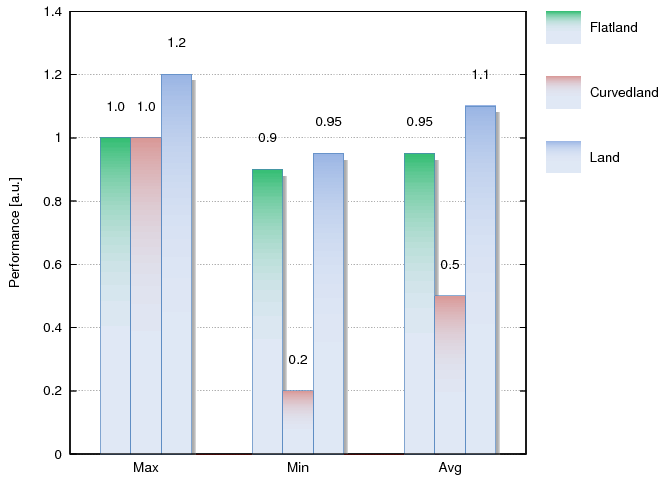# Historgrams

At the early stages of writing my blog on various gnuplot-related tricks, I discussed a way of making histograms a bit more interesting. The method was a bit awkward, for it relied on an external gawk script, but if you are interested, you can still check out the details.

However, in the course of time, I came to realise that there are easier ways of getting the same results, therefore, here I will only show the simpler route. But the problem of histograms is a bit broader than just making them shining. First we will look into how the histogram can be made horizontal.

## Turning a histogram

I have seen people searching for a solution to this problem with the histograms. If you needed it in the past, you have probably realised that gnuplot can make only vertical histograms. But sometimes, this is not the best way to represent data, and a horizontal version would be much better. For one thing, the horizontal one might take up much less space. If you want to learn how to produce the figure below, keep reading!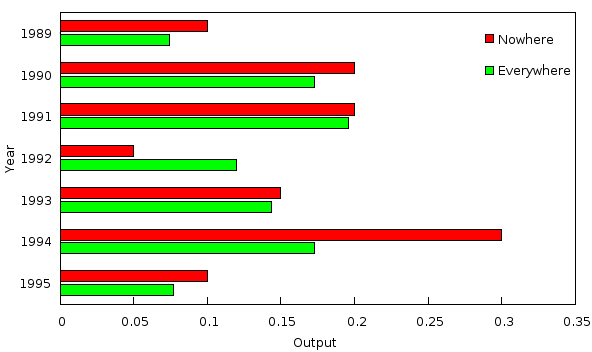Making the graphs will require some work, but everything is quite straightforward. What we should note, however, is that we will still produce an upright image that we have to rotate later. If you set the terminal to postscript, you will probably use the figure in LaTeX, where you can simply tell the compiler to rotate the figure by 90 degrees. If you set the terminal to a raster format, or print the screen, you can rotate the image in many applications, but if you want to adhere to the command line, you can, at least, under Linux, use the convert command as

```convert -rotate 90 figure_in.png figure_out.png
```

The figure above was produced based on the following data file

```1989 0.1
1990 0.2
1991 0.2
1992 0.05
1993 0.15
1994 0.3
1995 0.1
```

After this interlude, let us see the code! I will discuss it afterwards.

```reset
set key at graph 0.24, 0.85 horizontal samplen 0.1
set style data histogram
set style histogram cluster gap 1
set style fill solid border -1
set boxwidth 0.8
set xtic rotate by 90 scale 0
unset ytics
set y2tics rotate by 90
set yrange [0:0.35]; set xrange [-0.5:6.5]
set y2label 'Output' offset -2.5
set xlabel ' '
set size 0.6, 1
set label 1 'Year' at graph 0.5, -0.1 centre rotate by 180
set label 2 'Nowhere' at graph 0.09, 0.85 left rotate by 90
set label 3 'Everywhere' at graph 0.2, 0.85 left rotate by 90
p 'pie.dat' u 2 title ' ', '' u (\$2/2.0+rand(0)/10.0) title ' ', '' u 0:(0):xticlabel(1) w l title ''
```

The first line after the reset is required, because we have to make our key ourselves. We simply specify the coordinates and that that we want to have a horizontal key (i.e., one in which the keys are placed to the right of the previous one), with a length of 0.1. If you want smaller or larger space between the keys, you can modify this, by adding the spacing flag to the set command. You can see the exact syntax by issuing ?key in the gnuplot prompt. In the next four lines, we set up our histogram. The content of these lines might depend on what exactly we intend to plot. For more on this, check out the gnuplot demo page! We then rotate the xtics by 90 degrees. We do this, because the whole image will be rotated, and by rotating the xtics, we make sure that those will be horizontal at the end. For the very same reason, we also unset the ytics, and set the y2tics. We also set the y2label and xlabel. This latter one is empty, but we still need the space for it, so we set it to ' '. Beware the white space!

The next important step is the setting of the aspect ratio of our figure, which will be 0.6:1. Having done that, we introduce 3 labels: one for the xlabel, and two for the key. The placement of these labels is somewhat arbitrary, and depends on the particular terminal that you use. But only these three numbers and the coordinates of the key need any tweaking, really. At the very end, we plot our data. I plotted the second column twice, with an added random numbers, so that we can have two columns at each data point. Note that we plot the first column, too, but pass it to the xlabel command. By doing that, we can automate the labelling of the xtics, using the first column in our data file. Also note the specification of the titles: in the first two cases, the single quotes include a white space, while in the third one, there is nothing.

## Adding phong to a histogram

In certain cases, adding phong to any plotted object changes the whole character of the graph. The problem with them, at least, in gnuplot, is that adding the phong usually involves a lot of hassle, and external scripts. However, it turns out that many of these problems can be solved by a one-liner script. We will discuss a method of making shining histograms, without an external script, only with legal gnuplot commands, and in 5 lines. I understand that 5 lines is just 4 lines longer, than one would expect from a one-liner, but on the other hand, three out of those 5 lines are equivalent. So here is our figure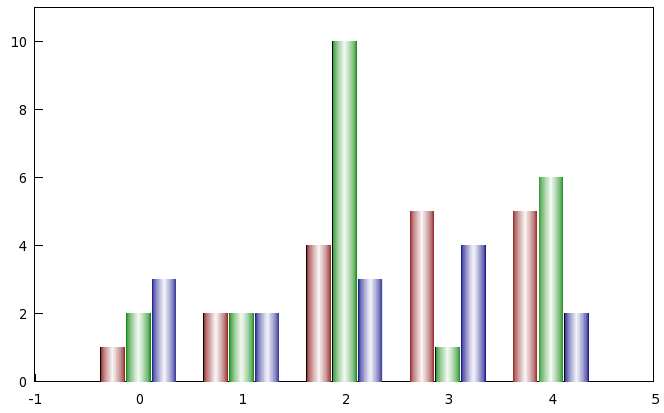here is our data file, which we will call 'hist.dat'

```1 2 3
2 2 2
4 10 3
5 1 4
5 6 2
```

and here are our scripts, 'hist.gnu',

```reset
unset key; set xtics nomirror; set ytics nomirror; set border front;
div=1.1; bw = 0.9; h=1.0; BW=0.9; wd=10; LIMIT=255-wd; white = 0
red = "#080000"; green = "#000800"; blue = "#000008"
set auto x
set yrange [0:11]
set style data histogram
set style histogram cluster gap 1
set style fill solid
set boxwidth bw
set multiplot
plot 'hist.dat' u 1 lc rgb red, '' u 2 lc rgb green, '' u 3 lc rgb blue
unset border; set xtics format " "; set ytics format " "; set ylabel " "
call 'hist_r.gnu'
unset multiplot
```

and 'hist_r.gnu'

```bw=BW*cos(white/LIMIT*pi/2.0); set boxwidth bw; white=white+wd
red = sprintf("#%02X%02X%02X", 128+white/2, white, white)
green = sprintf("#%02X%02X%02X", white, 128+white/2, white)
blue = sprintf("#%02X%02X%02X", white, white, 128+white/2)
rep
```

Then let us see what is happening here! At the beginning, we define various variables, most notably, white, red, green, and blue. The rest up to the first plot command is nothing but setting up the figure: we define the range, tell gnuplot to treat our data as histogram, set the width of the bars, and finally, set multiplot. There is nothing exciting in the first plot, except, that we specify the colour of the bars as

```plot 'hist.dat' u 1 lc rgb red, '' u 2 lc rgb green, '' u 3 lc rgb blue
```

The strings red, green, and blue were defined at the beginning of our first script, thus, we learn here that gnuplot will accept any defined (and valid) string as the specifier of the colour. After our first plot, we unset the border, re-set the format of the xtic and ytic to empty, and do likewise with the ylabel. Should there be an xlabel, we should have to do the same there. Having done this, we call our second script, which we will dissect now. This is really nothing but a 'for' loop, that we have discussed a couple of times before. In fact, quite a few times. The first two commands re-set the widths of the bars in the next plot. Note that I set the width in such a way that it would draw the outline of a circle as we step through the values of white. This is what we increment next, mind you!

The next three lines are basically identical: we re-define the colours, using a sprintf command in each step. If you recall how the RGB colours are defined, we have to create a string that looks like

```#00FF00
```

say. This is what our sprintf command will do, returning a string of this form that depends on the value of 'white'. There are some small nuances in the corresponding colour channel of red, green, and blue, respectively, namely, that the base colour for red was

```#080000
```

and we want to linearly interpolate between this colour, and white,

```FFFFFF
```

so, we have to apply the relevant linear function, but there is nothing beyond this. Obviously, if you are unhappy with the colour scheme that I have (I know full well that these are not the best colours...), this is the place where you would have to tamper with the script. When we are done with re-defining the widths, and the colours, we simply replot our histogram, and do that, as long as the value of white is smaller, than the limit that we set at the beginning. In this particular case, 245. In most cases, we do not need this many plots, by the way! For a raster plot, 10-12 steps, for a vector format, something like 15-16 steps should be more than enough.

At the end, we shouldn't forget about unsetting the multiplot. The only difficulty that I see with this figure is that it is not so straightforward to use a key. However, it is not terribly hard to come up with a solution for this problem: all we have to do is to put three vertical labels on the top of the first, second, and third column, indicating what they represent.

I should also point out that this scheme works for columnstacked histograms, too. We only have to replace the line

```set style histogram cluster gap 1
```

by

```set style histogram rowstacked
```

which produces the following figure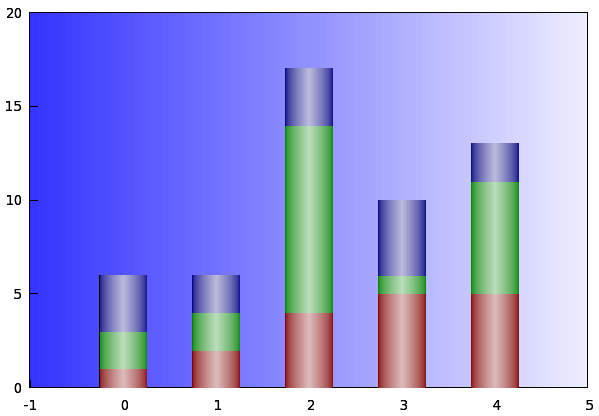## More complicated histograms

Someone asked me whether it would be possible to make a histogram that looks like those that MS Office can create: filled with gradient, casting a shadow, and labelled according to their value. Frankly, I just couldn't admit defeat, and I had to figure out a way. But then, it turned out to be rather simple, so I thought that I would share it here. We are going to make this figure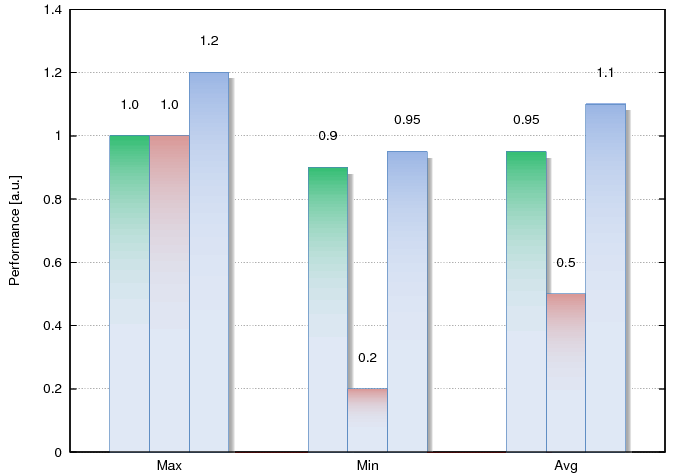from this data, called 'msbar.dat'

```Max 1.0 1.0 1.2
Min 0.9 0.2 0.95
Avg 0.95 0.5 1.1
```

We want to have gradients on the bars, thus, parametric plots would be the straightforward choice. However, using parametric, and then re-setting the palette each time can make the script rather convoluted. We can avoid that by plotting to a file first, and then plotting the file as many times as needed. We can't save the trouble of having to read our data file, but this is rather simple. We can do that either by employing a very primitive external script, or writing the script in gnuplot, as we discussed it in, say, the post on the recession graph. Given that we haven't got to process any data, this latter method is probably less desirous. Gnuplot is not for printing lines and the like, after all.

After the introduction, let us see the script!

```reset
# First, the gradient for the bars
set xrange [0:1]; set yrange [0:1]; set isosample 2, 200
set table 'msbar_bar.dat'
splot y
unset table```
```# Then we make the shadow
set isosample 200, 50
set table 'msbar_bar_sh.dat'
splot (1.0-exp((x-1.0)*20.)) #*(1.0-exp((y-1.0)*20.0))
unset table```
```reset
unset key; unset colorbox
xw = 0.2; set boxwidth xw; sh = 0.03
gf(x) = x*x*x*x change this, if a tighter gradient is needed
set cbrange [0:7]
set xrange [-0.5:2.5]
set yrange [0:1.4]
set grid ytics lw 0.5 lc rgb "#868686"
set xtics nomirror
set ylabel 'Performance [a.u.]'
set palette defined (0 'e0e8f5', 1 '#31bd71', 2 'e0e8f5', 3 'd99795', 4 'e0e8f5', 5 '#9ab5e4', 6 "#ffffff", 7 "#a2a2a2")
plot 'msbar.dat' u 0:(0):xticlabel(1) w l, \
'' u (\$0-xw):(\$2+0.1):(stringcolumn(2)) w labels, \
'' u (\$0):(\$3+0.1):(stringcolumn(3)) w labels, \
'' u (\$0+xw):(\$4+0.1):(stringcolumn(4)) w labels, \
'msbar_bar_sh.dat' u (\$1*xw-1.5*xw+sh):(\$2*1.0-sh):(\$3+6.0) w ima, \
'' u (\$1*xw-.5*xw+sh):(\$2*1.0-sh):(\$3+6.0) w ima, \
'' u (\$1*xw+.5*xw+sh):(\$2*1.2-sh):(\$3+6.0) w ima, \
'' u (\$1*xw-1.5*xw+sh+1):(\$2*0.9-sh):(\$3+6.0) w ima, \
'' u (\$1*xw-.5*xw+sh+1):(\$2*0.2-sh):(\$3+6.0) w ima, \
'' u (\$1*xw+.5*xw+sh+1):(\$2*0.95-sh):(\$3+6.0) w ima, \
'' u (\$1*xw-1.5*xw+sh+2):(\$2*0.95-sh):(\$3+6.0) w ima, \
'' u (\$1*xw-.5*xw+sh+2):(\$2*0.5-sh):(\$3+6.0) w ima, \
'' u (\$1*xw+.5*xw+sh+2):(\$2*1.1-sh):(\$3+6.0) w ima, \
'msbar_bar.dat' u (\$1*xw-1.5*xw):(\$2*1.0):(gf(\$3)) w ima, \
'' u (\$1*xw-.5*xw):(\$2*1.0):(gf(\$3)+2.0) w ima, \
'' u (\$1*xw+.5*xw):(\$2*1.2):(gf(\$3)+4.0) w ima, \
'' u (\$1*xw-1.5*xw+1):(\$2*0.9):(gf(\$3)) w ima, \
'' u (\$1*xw-.5*xw+1):(\$2*0.2):(gf(\$3)+2.0) w ima, \
'' u (\$1*xw+.5*xw+1):(\$2*0.95):(gf(\$3)+4.0) w ima, \
'' u (\$1*xw-1.5*xw+2):(\$2*0.95):(gf(\$3)) w ima, \
'' u (\$1*xw-.5*xw+2):(\$2*0.5):(gf(\$3)+2.0) w ima, \
'' u (\$1*xw+.5*xw+2):(\$2*1.1):(gf(\$3)+4.0) w ima, \
'msbar.dat' u (\$0-xw):2 w boxes lt -1 lw 0.5 lc rgb "#4f81bd", \
'' u (\$0):3 w boxes lt -1 lw 0.5 lc rgb "#4f81bd", \
'' u (\$0+xw):4 w boxes lt -1 lw 0.5 lc rgb "#4f81bd"
```

If you look at the graph, we have three different gradients, and the shadow. That makes four colour schemes altogether. Since we would like to save the difficulties associated with multiplot, we have to cram all those colour schemes into one colour range. More on this a bit later.

So, first we plot the gradient that will fill the bars, and then the "surface" that will represent our shadow. Then we set up our figure: we take off the keys, and unset the colour box. We also specify the width of our bars 'xw', and set the box width accordingly. This latter step is needed, because we want to have a border to the bars. The shadow shift, 'sh' is also defined here. In the next line, we set our colour range, in this particular case, between [0:7]. As we have pointed out, we need 4 colour ranges, and they should not overlap, therefore, we need 3 gaps between these ranges. For the sake of simplicity, we define the gap to be 1, and all the ranges to be one. This is why we end up with a total colour range of [0:7]. If you have more, or less bars to plot, you can re-define this range accordingly. The next couple of steps are trivial, up to the definition of the colour schemes. We want to have only simply gradients, thus, it is enough to define the colours at the end points. If you are unhappy with the colouring of your bars, you should change the colours here.

Having set up the figure, we can plot the data. First, we plot the labels for the xtics, and the values of the bars. Next comes the shadow. It might be a bit of an overkill for this figure, so you can skip all lines up to 'msbar_bar.dat'. Note that we simply go through all the data points in our file, and shift the shadow in each step. The height of the shadow is given by the product of the second column (which takes values between 0 and 1) of 'msbar_bar_sh.dat' and the particular data point in 'msbar.dat'. We also add a small downwards and rightwards shift to the shadows, lest they should be covered by the bars. The most important point, however, is that we plot the shadow as

```'' u (\$1*xw-.5*xw+sh):(\$2*1.0-sh):(\$3+6.0) w ima
```

i.e., the third column is shifted by 6. We do this, so that the shadow is pushed into the [6:7] range, where the shadow's colour scheme is defined. Plotting the bars happens in a similar fashion, the only difference is that we do not add 'sh' to the columns, and we push the values into the range of the appropriate colour range. At the very end, we plot the data file once more, this time with boxes, so that the bars can have a border to them.

If you want to use this plot many times, it might be worthwhile to implement it in a script in the language of your choice. The only thing required is printing lines and numbers. In pseudocode, it would look something like this:

```for i
for j
print "'msbar_bar_sh.dat' u (\$1*xw-(%d-1.5)*xw+sh):(\$2*%d-sh):(\$3+6.0) w ima, \", i, d
print "'msbar_bar.dat' u (\$1*xw-(%d-1.5)*xw):(\$2*%d):(\$3+%d) w ima, \", i, d, i
end
end
```

Now, suppose that you want to add a legend to the figure. The usual way, setting the key, will not work here, for obvious reasons. However, we can easily add that to the figure. We just have got to find some space for it. Since there is no space left on the figure, we have to make some: we will use multiplot, and specify the size of the main figure to be less, than 1. Therefore, adding the following lines to our gnu script should produce the legend.

```set multiplot
set size 0.8, 1
... (The main plot, identical to our original plot)
set origin 0.75, 0
unset border; unset xtics; unset ytics; unset ylabel; unset xlabel
set label 1 at first -0.15, 1.35 "Flatland"
set label 2 at first -0.15, 1.15 "Curvedland"
set label 3 at first -0.15, 0.95 "Land"
plot 'msbar_bar.dat' u (\$1*xw-0.4):(\$2/10.0+1.3):(gf(\$3)) w ima, \
'msbar_bar.dat' u (\$1*xw-0.4):(\$2/10.0+1.1):(gf(\$3)+2) w ima, \
'msbar_bar.dat' u (\$1*xw-0.4):(\$2/10.0+0.9):(gf(\$3)+4) w ima
unset multiplot
```

The script above results in the figure below: